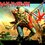# Frog on lotus leavesimg

$n(>1)$ lotus leaves are arranged in a circle.A frog jumps from a particular leaf by the following rule:It always moves counter clockwise.From starting point it skips one leaf and jumps to the next.Then it skips 2 leaves and jumps to the following.That is in $3$rd jump it skips $3$ leaves and in the $4$th jump it skips $4$ leaves and so on.In this manner it keeps moving round and round the circle of leaves.It may go to one leaf more than once.If it reaches each leaf at least once,then prove that $n$(The number of leaves) cannot be odd.

$\textbf{Note:}$A similar problem came in the entrance examination of the Indian Statistical Institute this year.7 years, 1 month ago

This discussion board is a place to discuss our Daily Challenges and the math and science related to those challenges. Explanations are more than just a solution — they should explain the steps and thinking strategies that you used to obtain the solution. Comments should further the discussion of math and science.

When posting on Brilliant:

• Use the emojis to react to an explanation, whether you're congratulating a job well done , or just really confused .
• Ask specific questions about the challenge or the steps in somebody's explanation. Well-posed questions can add a lot to the discussion, but posting "I don't understand!" doesn't help anyone.
• Try to contribute something new to the discussion, whether it is an extension, generalization or other idea related to the challenge.

MarkdownAppears as
*italics* or _italics_ italics
**bold** or __bold__ bold
- bulleted- list
• bulleted
• list
1. numbered2. list
1. numbered
2. list
Note: you must add a full line of space before and after lists for them to show up correctly
paragraph 1paragraph 2

paragraph 1

paragraph 2

[example link](https://brilliant.org)example link
> This is a quote
This is a quote
    # I indented these lines
# 4 spaces, and now they show
# up as a code block.

print "hello world"
# I indented these lines
# 4 spaces, and now they show
# up as a code block.

print "hello world"
MathAppears as
Remember to wrap math in $$ ... $$ or $ ... $ to ensure proper formatting.
2 \times 3 $2 \times 3$
2^{34} $2^{34}$
a_{i-1} $a_{i-1}$
\frac{2}{3} $\frac{2}{3}$
\sqrt{2} $\sqrt{2}$
\sum_{i=1}^3 $\sum_{i=1}^3$
\sin \theta $\sin \theta$
\boxed{123} $\boxed{123}$

Sort by:

Not just a similar problem, the same problem.

- 7 years, 1 month ago

I think they asked for somd minimum valurpe right? Thats why I said similar...

- 7 years, 1 month ago

Let no. Of leaves which frog jumped is x therefore no.of leaves left is equal to x(x+1)/2.so total no of leaves is x(x+3)/2...... This is where i reached if nyone canfurtur solve please help

- 7 years, 1 month ago

x

- 4 years, 7 months ago